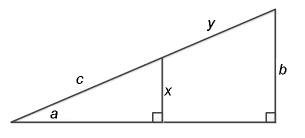Name:    Math 10W Practice Unit 5 Test #1

Multiple Choice
Identify the choice that best completes the statement or answers the question.

1.

A carpet has side lengths of 3.2 m and 4.6 m. What is the distance between opposite corners of the carpet?
 a. 3.3 m c. 5.6 m b. 2.8 m d. 7.9 m

2.

TV screen sizes are indicated by the distance between opposite corners. A big screen TV has sides that are 32 in high and 43 in wide. What is the size of the TV?
 a. 49 in c. 29 in b. 59 in d. 54 in

3.

Each side of a square is 10 cm long. What is the length of the diagonal of the square?
 a. 14.14 cm c. 17.14 cm b. 18.54 cm d. 20.14 cm

4.

What is an angle of depression?
 a. The angle between the horizon and the line of sight when looking down. b. The angle between the horizon and the line of sight when looking up. c. The angle between the hypotenuse and the vertical leg of a triangle. d. The angle between the vertical and horizontal legs of a triangle.

5.

A right triangle has an angle of 68° and the opposite side is 31 cm. What is the length of the hypotenuse?
 a. 12.52 cm c. 82.75 cm b. 33.43 cm d. 44.73 cm

6.

A right triangle has an angle of 15° and the opposite side is 28 cm. What is the length of the hypotenuse?
 a. 28.99 cm c. 104.50 cm b. 119.48 cm d. 108.18 cm

7.

A right triangle has an angle of 8° and the opposite side is 246 cm. What is the length of the hypotenuse?
 a. 248.42 cm c. 1750.38 cm b. 1778.88 cm d. 1767.58 cm

8.

A right triangle has an angle of 62°. If the opposite side is 57 cm long, what is the length of the adjacent side?
 a. 34.0 cm c. 30.3 cm b. 60.6 cm d. 64.6 cm

9.

Which side of a right triangle will be the longest if tan A is equal to 2?
 a. hypotenuse c. corresponding side b. opposite side d. adjacent side

10.

A right triangle has two sides measuring 10 m and 22 m, respectively. If one of the sides is the hypotenuse, what is the largest angle that can be between them?
 a. 27.0° c. 63.0° b. 24.4° d. 67.5°

1.

Will a pencil that is 15 cm long fit into a pencil case that is 13 cm long and 10 cm wide?

2.

A birdwatcher spots a bird that is perched on a branch 9.3 m off the ground. The angle of elevation is 21°. What is the shortest distance between the birdwatcher and the bird?

3.

The diagram below has the following dimensions:
a = 27°
b = 35 cm
c = 36 cm

What is the value of y?4.

A 8-m long extension cord is needed to power a drill that a worker is using at shoulder level, with the cord fully extended from the plug in the wall. The angle of elevation of the cord is 39.7°. What is the horizontal distance from the plug to where the worker is using the drill?

5.

A child rolls down a hill that is 6.7 m high. If he rolls a total of 11.8 m to reach the base of the hill, at what angle does the hill rise?